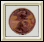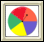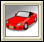How Likely Is It: Investigation 3: Making Decisions With Probability
 Arnold WorksiteStudent Resources Danicek WorksiteStudent Resources Grimmer WorksiteStudent Resources Homework Help Multiple-Choice Skills PracticeVocabulary Quiz Did You Know?Video Tutor Insert Video Links Here Pearson Video TutorCore Support Files

HOW LIKELY IS IT?

IXL Math

BBC Bitesize Math Probability ActivityCoin Tossing – Explore probability concepts by simulating repeated coin tosses.Hamlet Happens – Verify that rare events happen by drawing letters from a box.Spinners – Work with spinners to learn about numbers and probabilities.Stick or Switch – Investigate probabilities of sticking with a decision, or switching.Data Analysis & Probability Deal or No DealThe rules are simple. Choose a briefcase. Then as each round progresses, you must either stay with your original briefcase choice or make a "deal" with the bank to accept its cash offer in exchange for whatever dollar amount is in your chosen case.Data Analysis & Probability Guess the Number -1,000 to 1,000Guess a number from -1,000 to 1,000. What is the best strategy?Data Analysis & Probability Guess the Number 0 to 100Guess a number from 0 to 100. What is the best strategy?Data Analysis & Probability Probability - StarfishIt's a beautiful day at the beach. Let's learn about probability with starfish.Adjustable Spinner Creating a Spinner to Examine Experimental and Theoretical OutcomesFire Simulating the Spread of a Wildfire Using Probability
Create a game spinner with variable sized sectors to look at experimental and theoretical probabilities. Parameters: Sizes of sectors, number of sectors, number of trials.

Experiment with probability using a fixed size section spinner, a variable section spinner, two regular 6-sided dice or customized dice.

In this applet you can adjust the parameters on two Gaussian curves to determine if there is a possibility of a difference between the two means.

Create a game spinner with one to twelve sectors in order to look at experimental and theoretical probabilities. Parameters: Number of sectors, number of trials.

### How Likely Is It?

Concept with Explanation
Selected Homework from ACE

The unit How Likely Is It? was created to help students:

• Understand that probabilities are useful for predicting what will happen over the long run

• Understand the concepts of equally likely and not equally likely;

• Understand that a game of chance is fair only if each player has the same chance of winning, not just a possible chance of winning;

• Understand that there are two ways to build probability models: by gathering data from experiments (experimental probability) and by analyzing the possible equally likely outcomes (theoretical probability);

• Understand that experimental probabilities are better estimates of theoretical probabilities when they are based on larger numbers of trials;

• Develop strategies for finding both experimental and theoretical probabilities; and

• Critically interpret statements of probability to make decisions or answer questions.

 Vocabulary
 Picture Vocabuary Probability outcomes stem-and-leaf plot trial theoretical probability experimental probability outcomes simulation event trial tree diagram